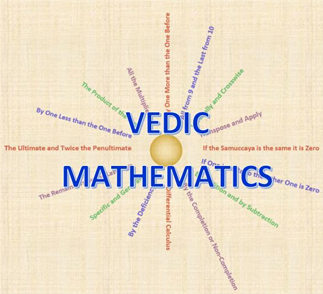# Rocket Maths

#### For Year 4 to Year 7

Rocket Maths is our fun and exciting 'Mental Math Technique Course' based on the system of Vedic Mathematics. Through the powerful Vedic System, our course will cover a broad range of topics ranging from basic arithmetic to complex algebraic equations.

The aim of the course is to develop a strong competency of mathematics, allowing students to solve difficult or large problems mentally with speed and efficiency. Altogether the Rocket Maths course aims to deliver a powerful intuition of mathematics to our students, which will benefit them in future academic studies.

For a student to be enrolled in the Rocket Maths Course, they must be between Year 4 and Year 8 . This is the prime age to acquire the benefits of Vedic Mathematics.

Rocket Maths is a 1 year long course and offers the complete mathematics package. It covers all branches of mathematics that the proposed age group is exposed to.

Brain Development and Mental Agility

Students will learn how to solve complex questions 'Mentally'. The brain is the only calculator pupils will need!

Creativity and Intuition

Rocket Maths equips pupils with a variety of problem solving techniques. The flexibility of the Vedic System means that students can even invent their own problem solving methods! Math becomes a creative and intuitive process.

Fast and Efficient

The simplicity of the techniques provided by our course means students can solve questions mentally quickly and efficiently!

Easy and Fun!

Rocket Maths is an intuitive and creative system. Students aren’t limited by the problem solving methods they can use. Math becomes a fun and exciting subject!

### The Power of Vedic Mathematics

Introduction: Vedic Mathematics: “Veda” means “knowledge”
(Courtesy: http://www.vedicmaths.org)Vedic Mathematics is an ancient system of mathematics originating in India. The system consists of a series of formulas (Sutras) that describe the way the mind naturally works. Vedic Maths is, therefore, a powerful system that directs students to solutions for various math problems.

Through the Vedic System, students are not limited to the one method. Instead, students are equipped with a variety of different methods which allow them to increase accuracy. Pupils can even invent their own methods, and in this way, mathematics becomes a creative and intuitive process

On top of this, difficult problems consisting of large sums are often solved immediately under the Vedic System. What’s more, calculations are mostly mental! The brain is the calculator.

 Our Rocket Maths course guides our pupils into the power of the Vedic System. By the end of the course, students are equipped with knowledge that applies to every branch of mathematics and will help them greatly in higher studies.

## Arithmetics, Squares and Square Roots

#### Rocket Maths 1.1

• In Rocket Maths 1.1 (RM1.1) students will master addition, subtraction, multiplication and division – the core foundation of mathematics. Students will learn how to solve complex calculations mentally and with speed through a variety of techniques.
• We’ll also introduce the concept of squaring and square roots through the unique and exclusive concept of the duplex.
• The aim of RM1.1 is to provide a comfortable yet engaging introduction to the Vedic system, a step towards developing a more creative, intuitive and fun attitude towards maths!

## Algebra, Fractions and Probability

#### Rocket Maths 1.2

• In Rocket Maths 1.2 (RM1.2), skills are developed further through more elaborate multiplication and division techniques which relate more to high school curricula
• Students are also introduced to algebra, learning how to construct algebraic equations from word problems and to solve various linear algebraic equations like algebraic fraction and division.
• This level will also develop and strengthen students’ knowledge of fractions and probability through various strategies unique to Vedic Maths.